# Running a Constrained Optimization With WeightsThis scenario shows you how to assign weights to constraints and how it can affect the geometry. The file provided is made up of a triangle. The scenario is divided into 2 steps:
• You first want to run the optimization so that the distance between the triangle vertex and Point.1 is as short as possible.
• Then you want to run the optimization so that the distance between the triangle vertex and Point.2 is as short as possible.

To do so, you define constraints and assign weights to these constraints.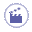1. Open the KwoConstraintswithWeights.CATPart file. The following image is displayed: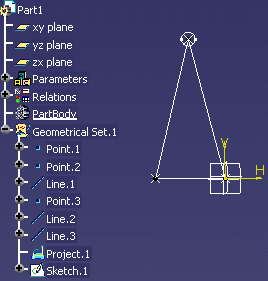This file contains a triangle. The sides of the triangle are assigned parameters valuated by formulas. f1: Distance between Point.1 and Point.3. It is valuated by Formula.1. f2: Distance between Point.2 and Point.3. It is valuated by Formula.2. l1: Distance between Point.1 and Point.3. It is valuated by Formula.3. l2: Distance between Point.2 and Point.3. It is valuated by Formula.4.
1. From the Start > Knowledgeware menu, access the Product Engineering Optimizer workbench and click the Optimize icon (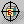). The Optimization dialog box is displayed.

2. Define the data required to run the optimization algorithm as follows:

### In the Problem tab

• In the Optimization type scrolling list, select the Only constraints option.

• Click Edit list in the Free Parameters field. Use the arrow key to select the following parameters and click OK when done:

 Geometrical Set.1\Point.3\X Geometrical Set.1\Point.3\Z
• In the Algorithm type scrolling list, select Simulated Annealing Algorithm.

### In the Constraints tab

• Click New... to create a constraint and enter the following script into the editor:

 `f1*f1==0`
• Click OK when done.

• Click New... to create a new constraint and enter the following script into the editor:

 `f2*f2==0`
• Click OK when done. The constraints are defined.

• Assign a weight to Constraint.1. To do so, click Constraint.1 in the table and enter 5 in the Weight field.

• Set the precision value to 0.01.

1. Click With update visualization and click Run optimization. Enter the name of the file that will contain the optimization data in the Save as dialog box: Constraintswithweights.xls, and click Save.

 The Optimization message box displays the data for each iteration. Do not intervene on the algorithm process (i.e. don't click Stop at the next iteration) and let the optimization process execute until the target value is found out or one of the termination condition is reached. The result is displayed in the Constraints tab.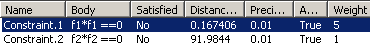The geometry is updated. The representation of Point.3 tends to the right. Point.3 coordinates are updated in the specification tree.
2. Assign a weight to Constraint.2. To do so, click Constraint.2 in the table and enter 10 in the Weight field.

3. Click Run optimization. Click Yes when prompted to overwrite Constraintswithweights.xls.

 The result is displayed in the Constraints tab.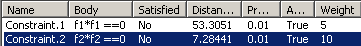The geometry is updated. The representation of Point.3 tends to the left. Point.3 coordinates are updated in the specification tree.
4. Click the Computations results tab. The results are sorted in the Sorted results table (see graphic below).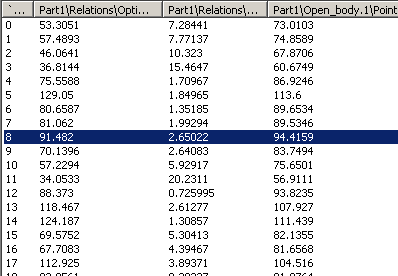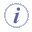Note that you can: